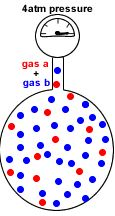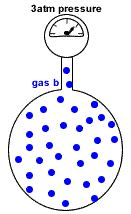# Dalton’s Law of Partial Pressures

Key Concepts

• Partial pressure of a gas in a mixture of gases is the pressure which that gas would exert if it were the only gas present in the container.
• Dalton’s Law of Partial Pressures states that the total pressure in a gas mixture is the sum of the partial pressures of each individual gas.

Ptotal = Pgas a + Pgas b + Pgas c + etc.

• Dalton’s Law of Partial Pressures assumes each gas in the mixture is behaving like an ideal gas.

Partial Pressure

A container of fixed volume at constant temperature holds a mixture of gas a and gas b at a total pressure of 4atm.
The total pressure in the container is proportional to the number of gas particles.

• More gas particles = greater pressure.
• Less gas particles = lower pressure.

If each dot represents 1 mole of gas particles, then there are 48 moles of gas particles in this container exerting a total pressure of 4 atm.Imagine the container with no particles of gas b.

Only particles of gas a are present in the same container at the same temperature.

Now the container holds only 12 moles of gas particles instead of the 48 moles of gas particles it originally contained.

Since pressure is proportional to the number of gas particles,  the pressure exerted by gas a = 12mol ÷ 48mol x 4atm = 1atmImagine the container with no particles of gas a.
Only particles of gas b are present in the same container at the same temperature.

Now the container holds only 36 moles of gas particles instead of the 48 moles of gas particles it originally contained.

Since pressure is proportional to the number of gas particles,  the pressure exerted by gas b = 36mol ÷ 48mol x 4atm = 3atm